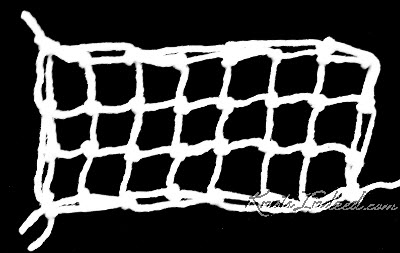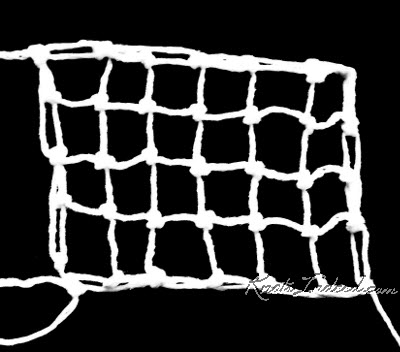Saturday, June 2, 2018

Rectangle of Square-Mesh – Generic Instructions #1 - Starting from the Middle

Once I had finished making rectangles for all four number of square variations  (even width with even length; odd width with odd length, even width with odd length, and odd width with even length) I checked to see if any of the instructions matched. To my delight, the instructions were the same for odd width with odd length and even width with odd length. Those rectangles which had even width with odd length, and odd width with even length used the same instructions. That meant there were only two different sets of generic instructions for rectangles of square-mesh that started from the middle of the rectangle.

The photos and instructions given below are for rectangles that have an even width and length or an odd width and length.Rectangle of Square-Mesh – 3 x 7 squares - Starting from the MiddleRectangle of Square-Mesh – 4 x 6 squares - Starting from the Middle

Here are my instructions for creating these pieces of square-mesh netting.

I made it with size 20 crochet thread, and used a #8 and a #6 knitting needle.

Before starting the actual netting:
1. Fill a netting needle or shuttle with thread or cord.
2. Attach it to a foundation loop.  To do this:
1. Tie a slip knot in the thread or cord coming from the needle or shuttle.  Leaving a tail, at least 2 to 3 inches.
2. Slip the foundation-loop cord through the slipknot.
3. Tie the foundation-loop cord into a circle thereby creating a foundation loop.
4. Tighten the slipknot.
3. Attach the foundation loop to a tension device.
4. Choose a mesh stick.

Selvage Edges - Generic Instructions (even width and length or odd width and length)
W = the number of squares desired in the width of the finished rectangle
L = the number of squares desired in the length of the finished rectangle

Start the Rectangle
Row 1: Using the small mesh stick, net as many more knots into the foundation loop as needed to obtain 1 more loop than you wish to have on each edge of the rectangle. (W + 1 loops in the row)
Remove the mesh stick and turn the work so that the next row can be worked from left to right. (This will be done at the end of each row.)
Row 2: Using the large mesh stick, net 1 knot in each loop. (W + 1 loops in the row)
Row 3: Using the large mesh stick, net 1 knot in each loop except the last 2 loops; net those last 2 loops together. (W loops in the row)
Continue by repeating Row 3 until there are only 2 loops in the row. (For each additional row, the number of loops will decrease by 1.)   When there are only 2 loops on a row, net those 2 loops together without using a mesh stick.
Cut the thread. Remove the netting from the foundation loop (which unties the knots created in Row 1) and undo the former knots in Row 1 to straighten the loops. Run the foundation-loop cord through one of the other rows of netting. Tie the thread from the netting needle to the loose thread at the end of Row 1.

Lengthen the Rectangle
1.       (Increase Row): Net 1 knot in each loop except the last loop; net 2 knots in the last loop.  (W + 2 loops in the row)
2.       (Decrease Row): Net 1 knot in each loop except the last loop; skip the last loop and turn the netting. (W + 1 loops in the row)
Repeat these 2 rows until the number of squares on the long side of the rectangle equals L – 1, OR until the number of rows equals L.

Finish the Rectangle
1.       (Decrease Row) Net 1 knot in each loop except the last 2 loops; net the last 2 loops together. (W loops in the row)
2.       Continue by repeating the decrease row until there are only 2 loops in the row. (For each additional row, the number of loops will decrease by 1.)   When there are only 2 loops on a row, net those 2 loops together without using a mesh stick. Cut the thread and remove the netting from the foundation loop.

1 comment:

blyskawica said...

Hi.